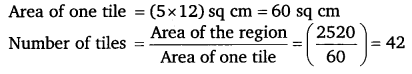NCERT Solutions for Class 6 Maths: One of the most important and interesting things to keep in mind is that these solutions available are totally free of cost. This also includes all the solutions to the exercises that are given in the textbook. NCERT solutions for class 6 maths gives you chapter-wise solutions to each and every question. This can help you solve even the tougher with ease.

It is always good to have a strong foundation in order to build a good building. This also is true in class when you are still learning the basics of maths. NCERT solutions for class 6 maths provides you the exact opportunity to build a strong foundation in this subject. Below is an overview of each and every chapter covered in the NCERT textbook.

## NCERT Solutions for Class 6 Maths Chapter 10 Mensuration

Ex 10.1 Class 6 Maths Question 1.
Find the perimeter of each of the following figures:Solution:
(a) Perimeter = the sum of the lengths of sides
= 5 cm +1 cm +2 cm + 4 cm = 12cm
(b) Perimeter = the sum of the lengths of sides
= 40 cm +35 cm + 23 cm +35 cm = 133 cm
(c) Perimeter = 4x the length of one side
= 4 x 15 cm = 60 cm
(d) Perimeter = 5 x the length of one side
4 = 5 x 4 cm = 20 cm
(e) Perimeter = the sum of the lengths of sides
= 4 cm + 0.5 cm + 2.5 cm + 2.5 cm + 0.5 cm + 4 cm + 1 cm = 15 cm
(f) Perimeter = the sum of the lengths of sides
= 4 cm + 3 cm + 2 cm + 3 cm + 1 cm + 4 cm + 3 cm + 2 cm + 3 cm + 1 cm + 4 cm + 3 cm + 2 cm +3 cm +1 cm + 4 cm + 3 cm + 2 cm + 3 cm + 1 cm = 52 cm

Ex 10.1 Class 6 Maths Question 2.
The lid of a rectangular box of sides 40 cm by 10 cm is sealed all round with tape. What is the length of the tape required?
Solution:
Length of the tape required
= Perimeter of the lid of a rectangular box = 2 x ( length + breadth)
= 2 x (40 cm +10 cm)
= 2 x 50 cm = 100 cm or 1 m

Ex 10.1 Class 6 Maths Question 3.
A table-top measures 2 m 25 cm by 1 m 50 cm. What is the perimeter of the table-top?
Solution:
Perimeter of the table-top
= 2 x (length + breadth)
= 2 x (2 m 25 cm +1 m 50 cm)
= 2 x (3 m 75 cm)
= 2 x 3.75 m = 7.50 m

Ex 10.1 Class 6 Maths Question 4.
What is the length of the wooden strip required to frame a photograph of length and breadth 32 cm and 21 cm, respectively?
Solution:
The length of the wooden strip required to frame a photograph is the perimeter of the photograph.
Perimeter of the photograph = 2 x (length + breadth)
= 2 x (32 cm +21 cm)
= 2 x 53 cm = 106 cm
∴ The length of the wooden strip required is 106 cm.

Ex 10.1 Class 6 Maths Question 5.
A rectangular piece of land measures 0.7 km by 0.5 km. Each side is to be fenced with 4 rows of wires. What is the length of the wire needed?
Solution:
We have to cover 4 times the perimeter of the land measuring 0.7 km by 0.5 km.
∴ Total length of wire required is 4 times its perimeter.
Perimeter of the land = 2 x (length + breadth)
= 2x (0.7 km+0.5 km)
= 2 x 1.2 km = 2.4 km
∴ Total length of wire required = 4 x 2.4 km = 9.6 km

Ex 10.1 Class 6 Maths Question 6.
Find the perimeter of each of the following shapes:
(a) A triangle of sides 3 cm, 4 cm and 5 cm.
(b) An equilateral triangle of side 9 cm.
(c) An isosceles triangle with equal sides 8 cm each and third side 6 cm.
Solution:
(a) Perimeter = the sum of the sides
= 3 cm + 4 cm + 5cm = 12 cm
(b) Perimeter = 3 x the length of one side
= 3 x 9 cm = 27 cm
(c) Perimeter = the sum of the lengths of the sides
= 8 cm + 8 cm + 6 cm = 22 cm

Ex 10.1 Class 6 Maths Question 7.
Find the perimeter of a triangle with sides measuring 10 cm, 14 cm and 15 cm.
Solution:
Perimeter of the triangle = the sum of the lengths of its sides
= 10 cm + 14 cm + 15 cm = 39 cm

Ex 10.1 Class 6 Maths Question 8.
Find the perimeter of a regular hexagon with each side measuring 8 m.
Solution:
A regular hexagon has 6 sides, so its perimeter
= 6 x length of its one side = 6 x 8 m
= 48 m

Ex 10.1 Class 6 Maths Question 9.
Find the side of the square whose perimeter is 20 m.
Solution:
Perimeter = 20 m
A square has 4 equal sides, so we can divide the perimeter by 4 to get the length of one side.
One side of the square = 20 m + 4 = 5m

Ex 10.1 Class 6 Maths Question 10.
The perimeter of a regular pentagon is 100 cm. How long is its each side?
Solution:
Perimeter = 100 cm
A regular pentagon has 5 equal sides, so we can divide the perimeter by
5 to get the length of one side
∴ length of one side = 100 cm + 5 = 20 cm

Ex 10.1 Class 6 Maths Question 11.
A piece of string is 30 cm long. What will be the length of each side if the string is used to forms
(a) a square?
(b) an equilateral triangle?
(c) a regular hexagon?
Solution:
(a) Perimeter = Length of the string = 30 cm
A square has 4 equal sides, so we can divide the perimeter by 4 to get the length of one side.
One side of the square = 30 cm + 4 = 7.5 cm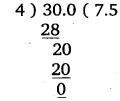(b)
Perimeter = Length of the string = 30 cm
An equilateral triangle has 3 equal sides, so we can divide the perimeter by 3 to get the length of one side.
∴ One side of an equilateral triangle = 30 cm ÷ 3 = 10 cm
(c) Perimeter = Length of the string = 30 cm
A regular hexagon has 6 equal sides, so we can divide the perimeter by
6 to get the length of one side.
One side of a regular hexagon = 30 cm + 6 = 5 cm

Ex 10.1 Class 6 Maths Question 12.
Two sides of a triangle are 12 cm and 14 cm. The perimeter of the triangle is 36 cm. What is its third side?
Solution:
Let ABC be the given triangle such that AB =12 cm, BC = 14 cm and its perimeter = 36 cm.
i.e., AB + BC + CA = 36 cm
or 12 cm + 14 cm + CA = 36 cm
or 26 cm + CA = 36 cm
or CA =36 cm – 26 cm = 10 cm
∴ The third side of triangle is 10 cm.

Ex 10.1 Class 6 Maths Question 13.
Find the cost offencing a square park ofside 250 mat the rate of ? 20 per metre.
Solution:
Side of the square park = 250 m
∴ Perimeter of the square park
= 4 x side
= 4 x 250 m = 1000 m
∴ Cost of fencing = ₹ (1000 x 20)
= ₹ 20000

Ex 10.1 Class 6 Maths Question 14.
Find the cost of fencing a rectangular park of length 175 m and breadth 125 m at the rate of? 12 per metre.
Solution:
Length of the rectangular park = 175 m Breadth of the rectangular park 125 m .-. Perimeter of the park = 2 x (length + breadth)
= 2 x (175m + 125m)
= 2 x 300 m = 600 m
∴ Cost of fencing = ₹ (600 x 12) = ₹ 7200

Ex 10.1 Class 6 Maths Question 15.
Sweety runs around a square park of side 75 m. Bulbul runs around a rectangular park with length 60 m and breadth 45 m. Who covers less distance?
Solution:
The distance each girl covers in one round is the same as the perimeter of the respective field. Therefore, the distance that Sweety covers in one round
= 4 x side
= 4 x 75 m = 300 m
Also, the distance that Bulbul covers in one round
= 2 x (length + breadth)
= 2 x (60 m + 45 m)
= 2 x 105 m = 210 m
This shows that Bulbul covers less distance than Sweety.

Ex 10.1 Class 6 Maths Question 16.
What is the perimeter of each of the following figures? What do you infer from the answer?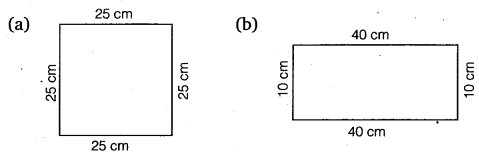Solution:
(a) Perimeter = 4 x side
= 4 x 25 cm
= 100 cm
(b) Perimeter = 2 x (length + breadth)
= 2 x (40 cm +10 cm)
= 2 x 50 cm = 100 cm
(c) Perimeter = 2 x (length + breadth)
2 x (30 cm +20 cm)
= 2 x 50 cm = 100 cm
(d) Perimeter = 30 cm +30 cm +40 cm = 100 cm
Thus, we observe that the perimeter of each figure is 100 cm i.e., they have equal perimeters.

Ex 10.1 Class 6 Maths Question 17.
Avneet buys 9 square paving slabs, each with a side of 1/2 m. He lays them in the form of a square.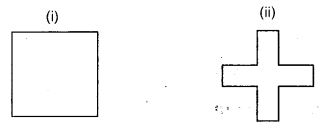(a)
What is the perimeter of his arrangement [Fig. (i)]?
(b) Shari does not like his arrangement. She gets him to lay them out like a cross. What is the perimeter of her arrangement [Fig. (ii)]?
(c) Which has greater perimeter?
(d) Avneet wonders if there is a way of getting an even greater perimeter. Can you find a way of doing this? (The paving slabs must meet along complete edges i.e. they cannot be broken.)
Solution:
(a) In case of Avneet’s arrangement:(b) In case of Shari’s arrangement: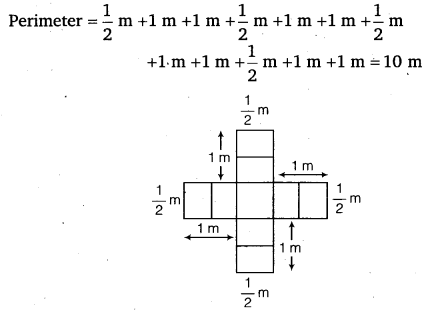(c) Clearly, perimeter in case of Shari is greater.
(d) Yes, there is a way shown in the figure in which we get a greater perimeterPerimeter = 2 x (9 + 1) units = 2 x 10 units = 20 units

Ex 10.2 Class 6 Maths Question 1.
Find the areas of the following figures by counting square: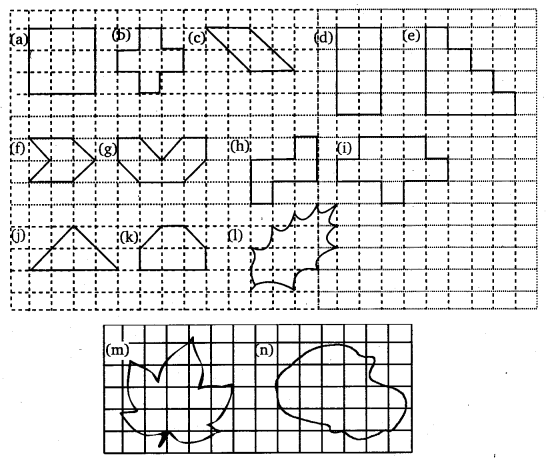Solution:
Placing these figures on the centimetre square, we have
(a) Full squares = 9
∴ Area covered by the figure = 9 x 1 sq. cm = 9 sq. cm

(b) Full squares = 5
∴ Area covered by the figure = 5 x 1 sq. cm = 5 sq. cm

(c) Full squares = 2
Half squares = 4
∴ Area covered by the figure (2×1+4×12) sq. cm
= (2 + 2) sq cm = 4 sq. cm

(d) Full squares = 10
∴ Area covered by the figure = 10 x 1 sq. cm = 10 sq. cm

(e) Full squares = 10
∴ Area covered by the figure = 10 x 1 sq. cm = 10 sq. cm

(f) Full squares = 2
Half squares = 4
∴ Area covered by the figure = (2×1+4×12) sq. cm
= (2 + 2)sq. cm = 4 sq. cm

(g) Full squares = 4
Half squares = 4
∴ Area covered by the figure = (2×1+4×12) sq. cm
= (4 + 2)sq. cm = 6 sq. cm

(h) Full squares = 5
∴ Area covered by the figure = 5 x 1 sq. cm
= 5 sq. cm

(i) Full squares = 9
∴ Area covered by the figure = 9 x 1 sq. cm
= 9 sq. cm

(j) Full squares = 2
Half squares = 4
∴ Area covered by the figure = (2×1+4×12) sq. cm
= (2 + 2)sq. cm = 4 sq. cm

(k) Full squares = 4
Half squares = 2
∴ Area covered by the figure = (4×1+2×12) sq. cm
= (4 + 1)sq. cm = 5 sq. cm

(l) Full squares = 4
More than half squares = 3
Half square = 2
∴ Area covered by the figure = (4×1+3×1+2×12) sq. cm
= (4 + 3 + 1)sq. cm
= 8 sq. cm

(m) Full squares = 7
More than half squares = 7
Half square = 0
∴ Area covered by the figure = (7×1+7×1+0×12) sq. cm
= (7 + 7 + 0)sq. cm
= 14 sq. cm

(n) Full squares = 10
More than half squares = 8
Half square = 0
∴ Area covered by the figure = (10×1+8×1+0×12) sq. cm
= (10 + 8 + 0)sq. cm
= 18 sq. cm

Ex 10.3 Class 6 Maths Question 1.
Find the areas of the rectangles whose sides are:
(a) 3 cm and 4 cm
(b) 12 m and 21 m
(c) 2 km and 3 km
(d) 2 m and 70 cm
Solution:
(a) Length of the rectangle = 4 cm
Breadth of the rectangle = 3 cm
= 4 cm x 3 cm
= 12 sq. cm

(b) Length of the rectangle = 21 m
Breadth of the rectangle = 12 m
= 21 m xl2 m
= 252 sq. m.

(c) Length of the rectangle = 3 km
Breadth of the rectangle =2 km
= 3 km x 2km
= 6 sq. km

(d) Length of the rectangle =2m = 2 x l00= 200 cm
Breadth of the rectangle = 70 cm
= 200 cm x 70 cm
= 14000 sq. cm

Ex 10.3 Class 6 Maths Question 2.
Find the areas of the squares whose sides are:
(a) 10 cm
(b) 14 cm
(c) 5 m
Solution:
(a) Side of the square = 10 cm
Area = (side)2
= (10)2 sq. cm
= 100 sq. cm

(b) Side of the square = 14 cm
Area = (side)2
= (14)2 sq. cm
= 196 sq. cm

(c) Side of the square = 5 m
Area = (side)2
= (5)2 sq. m = 25sq. m

Ex 10.3 Class 6 Maths Question 3.
The length and breadth of three rectangles are as given below:
(a) 9 m and 6 m
(b) 17 m and 3 m
(c) 4 m and 14 m
Which one has the largest area and which one has the smallest?
Solution:
In rectangle (a), Area = (9 x 6) sq. m
= 54 sq. m
In rectangle (b), Area =(3 x 17) sq. m = 51 sq. m
In rectangle (c), Area = (4 x 14) sq. m = 56 sq. m
Clearly, 56 > 54 > 51 i.e., 56 is the largest number and 51 is the smallest number.
∴ The rectangle (c) has the largest area and the rectangle (a) has the smallest area.

Ex 10.3 Class 6 Maths Question 4.
The area of a rectangular garden 50 m long is 300 sq m. Find the width of the garden.
Solution:
We use the formula A = l x b, where l is the length and b is the breadth of the rectangle in metre and its area in m2.
Here, A = 300 m2, and l = 50 m
Therefore, 300 =50 x b [ ∵ A = l x b]
or b=30050=6
Hence the breadth (width) of the rectangle is 6 m.

Ex 10.3 Class 6 Maths Question 5.
What is the cost of tiling a rectangular plot of land 500 m long and 200 m wide at the rate of ₹ 8 per hundred sq. m?
Solution:
Area of rectangular piece of land = 500 x 200 sq. m
= 100000 sq. m
Cost of tiling the land at the rate of ₹ 8 per hundred sq. m
= ₹ (100000×1100) = ₹ 8000

Ex 10.3 Class 6 Maths Question 6.
A table-top measures 2 m by 1 m 50 cm. What is its area in square metres?
Solution:
Length of table’s top = 2 m
Breadth of table’s top = 1 m 50 cm = 1.50 m
∴ Area = Length x Breadth
= (2 x 1.50) sq m = 3 sq m

Ex 10.3 Class 6 Maths Question 7.
A room is 4 m long and 3 m 50 cm wide. How many square metres of carpet is needed to cover the floor of the room?
Solution:
Length of the room = 4 m
Breadth of the room = 3 m 50 cm = 3.50 m
Carpet needed to cover the floor of the room
= Area of the floor of the room
= (4 x 3.50) sq. m = 14 sq. m

Ex 10.3 Class 6 Maths Question 8.
A floor is 5 m long and 4 m wide. A square carpet of sides 3 m is laid on the floor. Find the area of the floor that is not carpeted.
Solution: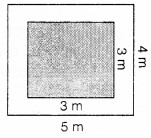Area of the floor of the room = (5 x 4) sq. m
= 20 sq. m
Area of the carpet = (3 x 3) sq. m = 9 sq. m
Area of the floor not carpeted = (20 – 9) sq. m
= 11 sq. m

Ex 10.3 Class 6 Maths Question 9.
Five square flower beds each of sides 1 m are dug on a piece of land 5 m long and 4 m wide. What is the area of the remaining part of the land?
Solution:
Area of the piece of land =(5 x 4) sq. m
= 20 sq. m
Area of square flower bed =(1 x 1) sq. m
= 1 sq. m
Area of 5 such flower beds =(5 x 1) sq. m
= 5 sq. m
∴ Area of the remaining part of land
= (20 – 5) sq. m = 15 sq. m

Ex 10.3 Class 6 Maths Question 10.
By splitting the following figures into rectangles, find their areas (The measures are given in centimetres).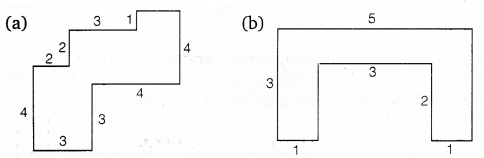Solution:
(a) Let the figure may be divided into rectangles marked as A, B, C etc. Area of rectangle (A) = (3 x 3) sq. cm = 9 sq. cmArea of rectangle (B) = (1 x 2) sq. cm = 2 sq. cm
Area of rectangle (C) = (3 x 3) sq. cm = 9 sq. cm
Area of rectangle (D) = (4 x 2) sq. cm = 8 sq. cm
∴ The total area of the figure = (9 + 2 + 9 + 8) sq. cm = 28 sq. cm
(b) Let the figure may be divided into rectangle marked as shown.Area of rectangle (A) = (2 x 1) sq cm = 2 sq cm
Area of rectangle (B) = (5 x 1) sq cm = 5 sq cm
Area of rectangle (C) = (2 x 1) sq cm = 2 sq cm
∴ Total area of the figure = (2 + 5 + 2) sq cm = 9 sq cm

Ex 10.3 Class 6 Maths Question 11.
Split the following shapes into rectangles and find their areas. (The measures are given in centimetres)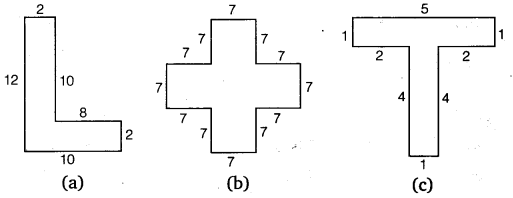Solution:
(a) Splitting the given shape into rectangles as marked A and B.Area of the rectangle (A) = (10 x 2) sq cm
= 20 sq cm
Area of the rectangle (B) = (10 x 2) sq cm
= 20 sq cm
∴ Total area of given shape = (20 +20) sq cm
= 40 sq cm
(b) Splitting the given shape into five squares each of side 7 cm.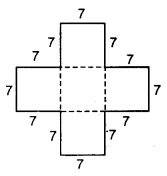Area of the given shape
= 5 x Area of one square of side 7 cm = 5 x (7 x 7) sq. cm
= (5 x 49)sq cm 7
= 245 sq cm
(c) Splitting the given shape into two rectangles named A and B. Area of rectangle (A) = (5 x 1) sq cm = 5 sq cm
Area of rectangle (B) = (4 x 1) sq cm = 4 sq cm
∴ Total area of given shape = (5 + 4) sq cm = 9 sq cm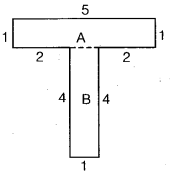Ex 10.3 Class 6 Maths Question 12.
How many tiles whose length and breadth are 12 cm and 5 cm, respectively will be needed to fit in a rectangular region whose length and breadth are respectively:
(a) 100 cm and 144 cm
(b) 70 cm and 36 cm
Solution:
(a) Area of the rectangular region
= (100 x 144) sq cm
= 14400 sq cm
Area of one tile = (5 x 12) sq cm
= 60 sq cm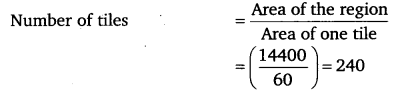(b) Area of the rectangular region
= (70 x 36) sq cm
= 2520 sq cm